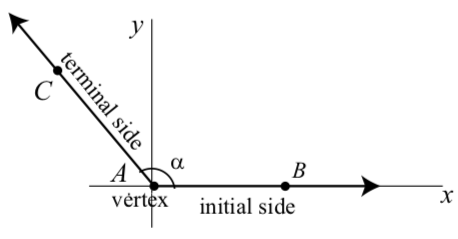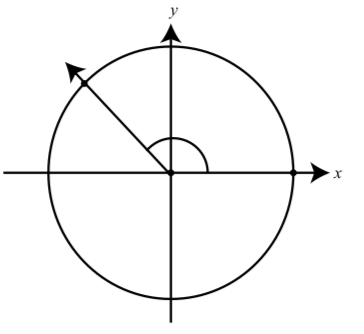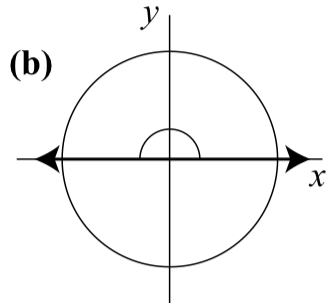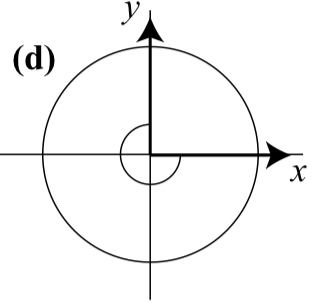$$\newcommand{\id}{\mathrm{id}}$$ $$\newcommand{\Span}{\mathrm{span}}$$ $$\newcommand{\kernel}{\mathrm{null}\,}$$ $$\newcommand{\range}{\mathrm{range}\,}$$ $$\newcommand{\RealPart}{\mathrm{Re}}$$ $$\newcommand{\ImaginaryPart}{\mathrm{Im}}$$ $$\newcommand{\Argument}{\mathrm{Arg}}$$ $$\newcommand{\norm}{\| #1 \|}$$ $$\newcommand{\inner}{\langle #1, #2 \rangle}$$ $$\newcommand{\Span}{\mathrm{span}}$$

# 1.3: Arcs, Angles, and Calculators

$$\newcommand{\vecs}{\overset { \rightharpoonup} {\mathbf{#1}} }$$ $$\newcommand{\vecd}{\overset{-\!-\!\rightharpoonup}{\vphantom{a}\smash {#1}}}$$$$\newcommand{\id}{\mathrm{id}}$$ $$\newcommand{\Span}{\mathrm{span}}$$ $$\newcommand{\kernel}{\mathrm{null}\,}$$ $$\newcommand{\range}{\mathrm{range}\,}$$ $$\newcommand{\RealPart}{\mathrm{Re}}$$ $$\newcommand{\ImaginaryPart}{\mathrm{Im}}$$ $$\newcommand{\Argument}{\mathrm{Arg}}$$ $$\newcommand{\norm}{\| #1 \|}$$ $$\newcommand{\inner}{\langle #1, #2 \rangle}$$ $$\newcommand{\Span}{\mathrm{span}}$$ $$\newcommand{\id}{\mathrm{id}}$$ $$\newcommand{\Span}{\mathrm{span}}$$ $$\newcommand{\kernel}{\mathrm{null}\,}$$ $$\newcommand{\range}{\mathrm{range}\,}$$ $$\newcommand{\RealPart}{\mathrm{Re}}$$ $$\newcommand{\ImaginaryPart}{\mathrm{Im}}$$ $$\newcommand{\Argument}{\mathrm{Arg}}$$ $$\newcommand{\norm}{\| #1 \|}$$ $$\newcommand{\inner}{\langle #1, #2 \rangle}$$ $$\newcommand{\Span}{\mathrm{span}}$$

Focus Questions

After studying this section, we should understand the concepts motivated by these questions and be able to write precise, coherent answers to these questions.

• How do we measure angles using degrees?
• What do we mean by the radian measure of an angle? How is the radian measure of an angle related to the length of an arc on the unit circle?
• Why is radian measure important?
• How do we convert from radians to degrees and from degrees to radians?
• How do we use a calculator to approximate values of the cosine and sine functions?

The ancient civilization known as Babylonia was a cultural region based in southern Mesopotamia, which is present-day Iraq. Babylonia emerged as an independent state around 1894 BCE. The Babylonians developed a system of mathematics that was based on a sexigesimal (base 60) number system. This was the origin of the modern day usage of 60 minutes in an hour, 60 seconds in a minute, and 360 degrees in a circle.

Many historians now believe that for the ancient Babylonians, the year consisted of 360 days, which is not a bad approximation given the crudeness of the ancient astronomical tools. As a consequence, they divided the circle into 360 equal length arcs, which gave them a unit angle that was 1/360 of a circle or what we now know as a degree. Even though there are 365.24 days in a year, the Babylonian unit angle is still used as the basis for measuring angles in a circle. Figure $$\PageIndex{1}$$ shows a circle divided up into 6 angles of 60 degrees each, which is also something that fit nicely with the Babylonian base-60 number system.Figure $$\PageIndex{1}$$: A circle with six 60-degree angles.

We often denote a line that is drawn through 2 points A and B by $$\overleftrightarrow{AB}$$. The portion of the line $$\overleftrightarrow{AB}$$ that starts at the point A and continues indefinitely in the direction point of point B is called ray AB and is denoted by $$\overrightarrow{AB}$$. The point A is the initial point of ray $$\overrightarrow{AB}$$. An angle is formed by rotating a ray about its endpoint. The ray in its initial position is called the initial side of the angle, and the position of the ray after it has been rotated is called the terminal side of the ray. The endpoint of the ray is called the vertex of the angle.Figure $$\PageIndex{2}$$: An angle including some notation.

Figure $$\PageIndex{2}$$ shows the ray $$\overrightarrow{AB}$$ rotated about the point A to form an angle. The terminal side of the angle is the ray $$\overrightarrow{AC}$$. We often refer to this as angle BAC, which is abbreviated as $$\angle{BAC}$$. We can also refer to this angle as angle $$CAB$$ or $$\angle{CAB}$$. If we want to use a single letter for this angle, we often use a Greek letter such as $$\alpha$$(alpha). We then just say the angle ̨. Other Greek letters that are often used are $$\beta$$(beta), $$\gamma$$(gamma), $$\theta$$(theta), $$\phi$$(phi), and $$\rho$$(rho).

## Arcs and Angles

To define the trigonometric functions in terms of angles, we will make a simple connection between angles and arcs by using the so-called standard position of an angle. When the vertex of an angle is at the origin in the $$xy$$-plane and the initial side lies along the positive x-axis, we see that the angle is in standard position. The terminal side of the angle is then in one of the four quadrants or lies along one of the axes. When the terminal side is in one of the four quadrants, the terminal side determines the so-called quadrant designation of the angle. See Figure $$\PageIndex{3}$$.Figure $$\PageIndex{3}$$: Standard position of an angle in the second quadrant.

Exercise $$\PageIndex{1}$$

Draw an angle in standard position in

These graphs show positive angles in standard position. The one on the left has its terminal point in the first quadrant, the one in the middle has its terminal point in the third quadrant, and the one on the right has its terminal point in the fourth quadrant.If an angle is in standard position, then the point where the terminal side of the angle intersects the unit circle marks the terminal point of an arc as shown in Figure 1.11. Similarly, the terminal point of an arc on the unit circle determines a ray through the origin and that point, which in turn defines an angle in standard position. In this case we say that the angle is subtended by the arc. So there is a natural correspondence between arcs on the unit circle and angles in standard position. Because of this correspondence, we can also define the trigonometric terminal side functions in terms of angles as well as arcs. Before we do this, however, we need to discuss two different ways to measure angles.Figure $$\PageIndex{4}$$: An arc and its corresponding angle.

There are two ways we will measure angles – in degrees and radians. When we measure the length of an arc, the measurement has a dimension (the length, be it inches, centimeters, or something else). As mentioned in the introduction, the Babylonians divided the circle into 360 regions. So one complete wrap around a circle is 360 degrees, denoted $$360^\circ$$. The unit measure of $$1^\circ$$ is an angle that is 1/360 of the central angle of a circle. Figure $$\PageIndex{1}$$ shows 6 angles of $$60^\circ$$ each. The degree $$^\circ$$ is a dimension, just like a length. So to compare an angle measured in degrees to an arc measured with some kind of length, we need to connect the dimensions. We can do that with the radian measure of an angle.

Radians will be useful in that a radian is a dimensionless measurement. We want to connect angle measurements to arc measurements, and to do so we will directly define an angle of 1 radian to be an angle subtended by an arc of length 1 (the length of the radius) on the unit circle as shown in Figure $$\PageIndex{5}$$.Figure $$\PageIndex{5}$$: One radian.

An angle of one radian is the angle in standard position on the unit circle that is subtended by an arc of length 1 (in the positive direction).

This directly connects angles measured in radians to arcs in that we associate a real number with both the arc and the angle. So an angle of 2 radians cuts off an arc of length 2 on the unit circle, an angle of 3 radians cuts of an arc of length 3 on the unit circle, and so on. Figure 1.13 shows the terminal sides of angles with measures of 0 radians, 1 radian, 2 radians, 3 radians, 4 radians, 5 radians, and 6 radians. Notice that $$2\pi \approx 6.2832$$ and so $$6 < 2\pi$$ as shown in Figure $$\PageIndex{6}$$.Figure $$\PageIndex{6}$$: Angles with Radian Measure 1, 2, 3, 4, 5, and 6

We can also have angles whose radian measure is negative just like we have arcs with a negative length. The idea is simply to measure in the negative (clockwise) direction around the unit circle. So an angle whose measure is $$-1$$ radian is the angle in standard position on the unit circle that is subtended by an arc of length 1 in the negative (clockwise) direction.

So in general, an angle (in standard position) of t radians will correspond to an arc of length t on the unit circle. This allows us to discuss the sine and cosine of an angle measured in radians. That is, when we think of sin$$(t)$$ and cos$$(t)$$, we can consider $$t$$ to be:

• a real number;
• the length of an arc with initial point $$(1, 0)$$ on the unit circle;
• the radian measure of an angle in standard position.

When we draw a picture of an angle in standard position, we often draw a small arc near the vertex from the initial side to the terminal side as shown in Figure $$\PageIndex{7}$$, which shows an angle whose measure is $$\dfrac{3}{4}\pi$$ radians.

Exercise $$\PageIndex{2}$$

1. Draw an angle in standard position with a radian measure of:Figure $$\PageIndex{7}$$: An angle with measure $$\dfrac{3}{4}\pi$$ in standard position

1. $$\dfrac{\pi}{2}$$ radians.
2. $$\pi$$ radians.
3. $$\dfrac{3\pi}{2}$$ radians.
4. $$\dfrac{-3\pi}{2}$$ radians.

2. What is the degree measure of each of the angles in part (1)?

1.2. $90^\circ$
3. $180^\circ$
4. $270^\circ$
5. $-270^\circ$

## Conversion Between Radians and Degrees

Radian measure is the preferred measure of angles in mathematics for many reasons, the main one being that a radian has no dimensions. However, to effectively use radians, we will want to be able to convert angle measurements between radians and degrees.

Recall that one wrap of the unit circle corresponds to an arc of length $$2\pi$$, and an arc of length $$2\pi$$ on the unit circle corresponds to an angle of $$2\pi$$ radians. An angle of $$360^\circ$$ is also an angle that wraps once around the unit circle, so an angle of $$360^\circ$$ is equivalent to an angle of $$2\pi$$ radians, or

• each degree is $$\dfrac{\pi}{180}$$ radians,
• each radian is $$\dfrac{180}{\pi}$$ degrees.

Notice that 1 radian is then $$\dfrac{180}{\pi} \approx 57.3^\circ$$, so a radian is quite large compared to a degree. These relationships allow us to quickly convert between degrees and radians.

Example $$\PageIndex{1}$$

• If an angle has a degree measure of 35 degrees, then its radian measure can be calculated as follows:

$35 \space degrees \times \dfrac{\pi \space radians}{180 \space degrees} = \dfrac{35\pi}{180} \space radians$

Rewriting this fraction, we see that an angle with a measure of 35 degrees has a radian measure of $$\dfrac{7\pi}{36}$$ radians.

• If an angle has a radian measure of $$\dfrac{3\pi}{10}$$ radians, then its degree measure can be calculated as follows:

$\dfrac{3\pi}{10} \space radians \space \times \dfrac{180 \space degrees}{\pi \space radians}= \dfrac{540}{10} \space degrees$

So an angle with a radian measure of $$\dfrac{3\pi}{10}$$ has an angle measure of 54$$^\circ$$.

IMPORTANT NOTE

Since a degree is a dimension, we MUST include the degree mark $$^\circ$$ whenever we write the degree measure of an angle. A radian has no dimension so there is no dimension mark to go along with it. Consequently, if we write 2 for the measure of an angle we understand that the angle is measured in radians. If we really mean an angle of 2 degrees, then we must write 2$$^\circ$$.

Exercise $$\PageIndex{3}$$

Complete the following table to convert from degrees to radians and vice versa.

Angle in Radians Angle in degrees
$$0$$ $$0^\circ$$
$$\dfrac{\pi}{6}$$ $$30^\circ$$
$$\dfrac{\pi}{4}$$ $$45^\circ$$
$$\dfrac{\pi}{3}$$ $$60^\circ$$
$$\dfrac{\pi}{2}$$ $$90^\circ$$
$$\dfrac{7\pi}{6}$$ $$210^\circ$$
$$\dfrac{5\pi}{4}$$ $$225^\circ$$
$$\dfrac{4\pi}{3}$$ $$240^\circ$$
$$\dfrac{3\pi}{2}$$ $$270^\circ$$
$$\dfrac{5\pi}{3}$$ $$300^\circ$$
$$\dfrac{2\pi}{3}$$ $$120^\circ$$
$$\dfrac{4\pi}{3}$$ $$135^\circ$$
$$\dfrac{5\pi}{6}$$ $$150^\circ$$
$$\pi$$ $$180^\circ$$
$$\dfrac{7\pi}{4}$$ $$315^\circ$$
$$\dfrac{11\pi}{6}$$ $$330^\circ$$
$$2\pi$$ $$360^\circ$$

## Calculators and the Trigonometric Functions

We have now seen that when we think of $$\sin(t)$$ or $$\cos(t)$$, we can think of $$(t)$$ as a real number, the length of an arc, or the radian measure of an angle. In Section 1.5, we will see how to determine the exact values of the cosine and sine functions for a few special arcs (or angles). For example, we will see that $$\cos(\dfrac{\pi}{6}) = \dfrac{\sqrt{3}}{2}$$. However, the definition of cosine and sine as coordinates of points on the unit circle makes it difficult to find exact values for these functions except at very special arcs. While exact values are always best, technology plays an important role in allowing us to approximate the values of the circular (or trigonometric)functions. Most hand-held calculators, calculators in phone or tablet apps, and online calculators have a cosine key and a sine key that you can use to approximate values of these functions, but we must keep in mind that the calculator only provides an approximation of the value, not the exact value (except for a small collection of arcs). In addition, most calculators will approximate the sine and cosine of angles.

 Angle in radians Angle in degrees 0 $$0^\circ$$ $$\dfrac{\pi}{6}$$ $$\dfrac{\pi}{4}$$ $$\dfrac{\pi}{3}$$ $$\dfrac{\pi}{2}$$ $$90^\circ$$ $$120^\circ$$ $$\dfrac{3\pi}{4}$$ $$135^\circ$$ $$150^\circ$$ $$180^\circ$$ $$\dfrac{7\pi}{6}$$ $$\dfrac{5\pi}{4}$$ $$\dfrac{4\pi}{3}$$ $$\dfrac{3\pi}{2}$$ $$270^\circ$$ $$300^\circ$$ $$315^\circ$$ $$330^\circ$$ $$2\pi$$ $$360^\circ$$

Table 1.1: Conversions between radians and degrees.

To do this, the calculator has two modes for angles: Radian and Degree. Be- cause of the correspondence between real numbers, length of arcs, and radian measures of angles, for now, we will always put our calculators in radian mode. In fact, we have seen that an angle measured in radians subtends an arc of that radian measure along the unit circle. So the cosine or sine of an angle measure in radians is the same thing as the cosine or sine of a real number when that real number is interpreted as the length of an arc along the unit circle. (When we study the trigonometry of triangles in Chapter 3, we will use the degree mode. For an introductory discussion of the trigonometric functions of an angle measure in degrees, see Exercise (4)).

Exercise $$\PageIndex{4}$$

In Exercise 1.6, we used the Geogebra Applet called Terminal Points of Arcs on the Unit Circle at http://gvsu.edu/s/JY to approximate the values of the cosine and sine functions at certain values. For example, we found that

• $$\cos(1) \approx 0.5403$$, $$\sin(1) \approx 0.8415$$.
• $$\cos(2) \approx -0.4161$$, $$\sin(2) \approx 0.9093$$.
• $$\cos(-4) \approx -0.6536$$, $$\sin(-4) \approx 0.7568$$.
• $$\cos(-15) \approx -0.7597$$, $$\sin(-15) \approx -0.6503$$.

Use a calculator to determine these values of the cosine and sine functions and compare the values to the ones above. Are they the same? How are they different?Using a calculator, we obtain the following results correct to ten decimal places.

$$\cos(1) \approx 0.5403023059, \sin(1) \approx 0.8414709848$$. $$\cos(1) \approx 0:4161468365, \sin(1) \approx 0.9092974268$$.

The difference between these values and those obtained in Progress Check 1.6 is that these values are correct to 10 decimal places (and the others are correct to 4 decimal places). If we round off each of the values above to 4 decimal places, we get the same results we obtained in Progress Check 1.6.

• $$\cos(1) \approx 0:6536436209, \sin(1) \approx 0.7568024953$$.
• $$\cos(1) \approx 0:7596879129, \sin(1) \approx 0.6502878402$$.

Summary of Section 1.3

In this section, we studied the following important concepts and ideas:

• An angle is formed by rotating a ray about its endpoint. The ray in its initial position is called the initial side of the angle, and the position of the ray after it has been rotated is called the terminal side of the ray. The endpoint of the ray is called the vertex of the angle.
• When the vertex of an angle is at the origin in the $$xy$$-plane and the initial side lies along the positive x-axis, we see that the angle is in standard position.
• There are two ways to measure angles. For degree measure, one complete wrap around a circle is 360 degrees, denoted 360$$^\circ$$. The unit measure of 1$$^\circ$$ is an angle that is 1=360 of the central angle of a circle. An angle of one radian is the angle in standard position on the unit circle that is subtended by an arc of length 1 (in the positive direction).
• We convert the measure of an angle from degrees to radians by using the fact that each degree is radians. We convert the measure of an angle from 180 radians to degrees by using the fact that each radian is 180 degrees.

1.3: Arcs, Angles, and Calculators is shared under a CC BY-NC-SA 3.0 license and was authored, remixed, and/or curated by Ted Sundstrom & Steven Schlicker via source content that was edited to conform to the style and standards of the LibreTexts platform; a detailed edit history is available upon request.